## Types of Graphs-

Before you go through this article, make sure that you have gone through the previous article on various Types of Graphs in Graph Theory.

We have discussed-

• A graph is a collection of vertices connected to each other through a set of edges.
• The study of graphs is known as Graph Theory.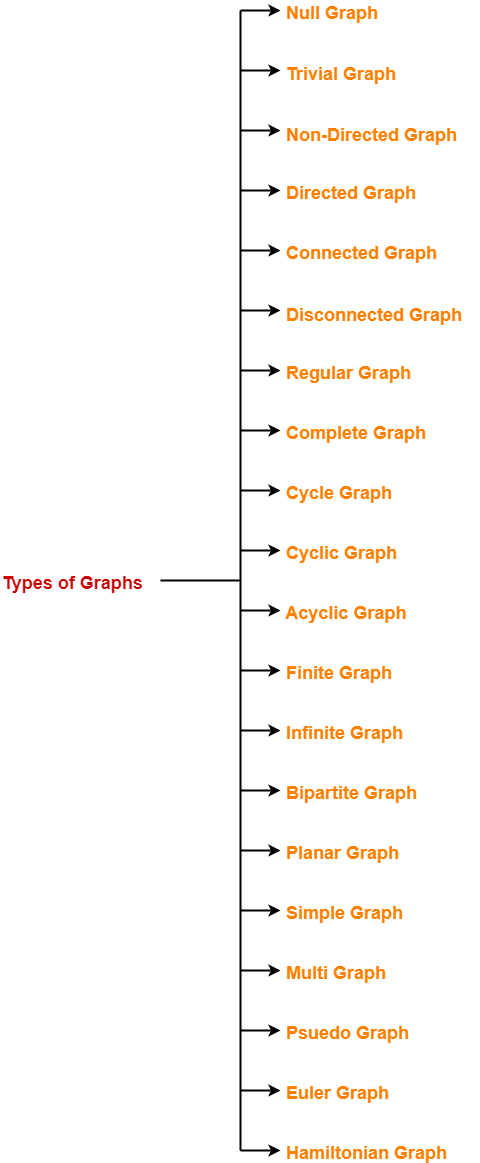## Euler Graph-

An Euler graph may be defined as-

 Any connected graph is called as an Euler Graph if and only if all its vertices are of even degree.ORAn Euler Graph is a connected graph that contains an Euler Circuit.

### Euler Graph Example-

The following graph is an example of an Euler graph-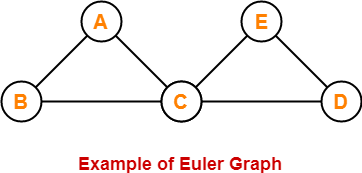Here,

• This graph is a connected graph and all its vertices are of even degree.
• Therefore, it is an Euler graph.

Alternatively, the above graph contains an Euler circuit BACEDCB, so it is an Euler graph.

## Euler Path-

Euler path is also known as Euler Trail or Euler Walk.

• If there exists a Trail in the connected graph that contains all the edges of the graph, then that trail is called as an Euler trail.

OR

• If there exists a walk in the connected graph that visits every edge of the graph exactly once with or without repeating the vertices, then such a walk is called as an Euler walk.

### NOTE

A graph will contain an Euler path if and only if it contains at most two vertices of odd degree.

### Euler Path Examples-

Examples of Euler path are as follows-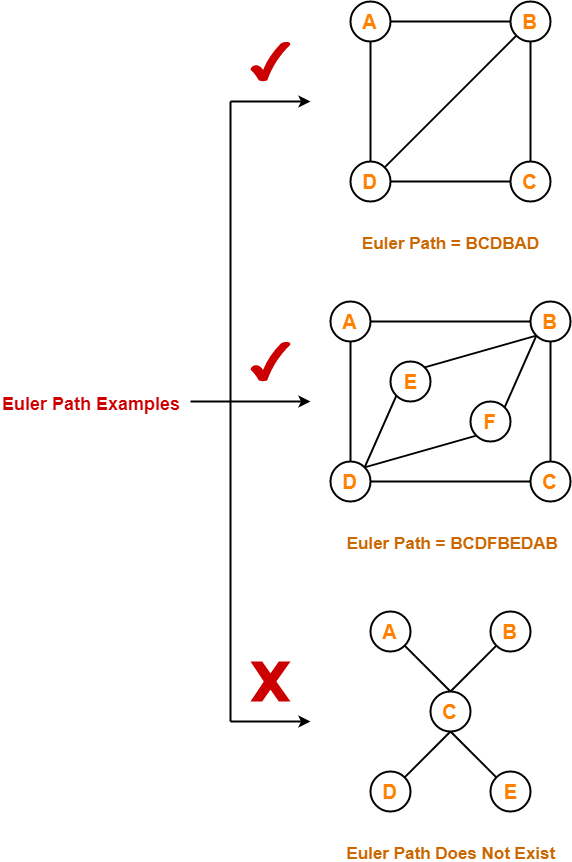## Euler Circuit-

Euler circuit is also known as Euler Cycle or Euler Tour.

• If there exists a Circuit in the connected graph that contains all the edges of the graph, then that circuit is called as an Euler circuit.

OR

• If there exists a walk in the connected graph that starts and ends at the same vertex and visits every edge of the graph exactly once with or without repeating the vertices, then such a walk is called as an Euler circuit.

OR

• An Euler trail that starts and ends at the same vertex is called as an Euler circuit.

OR

• A closed Euler trail is called as an Euler circuit.

### NOTE

A graph will contain an Euler circuit if and only if all its vertices are of even degree.

### Euler Circuit Examples-

Examples of Euler circuit are as follows-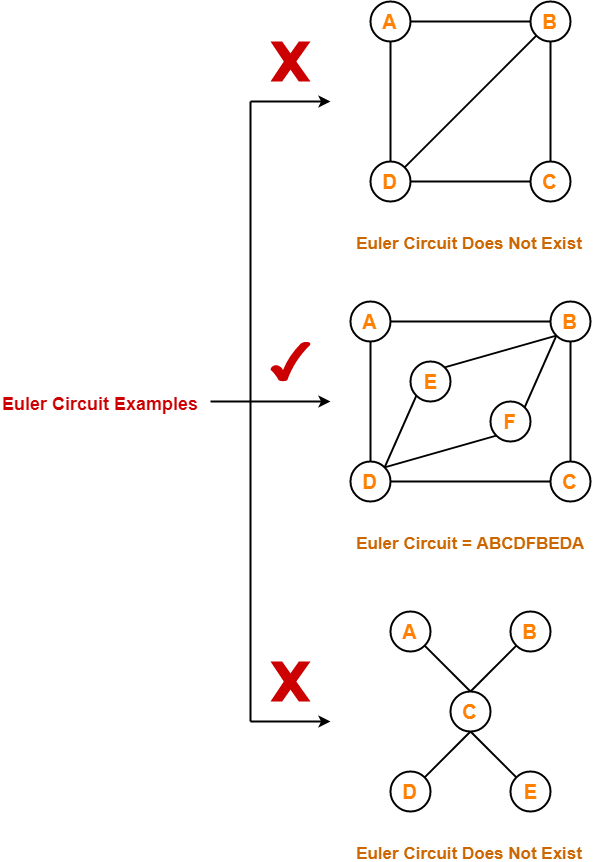## Semi-Euler Graph-

If a connected graph contains an Euler trail but does not contain an Euler circuit, then such a graph is called as a semi-Euler graph.

Thus, for a graph to be a semi-Euler graph, following two conditions must be satisfied-

• Graph must be connected.
• Graph must contain an Euler trail.

### Example-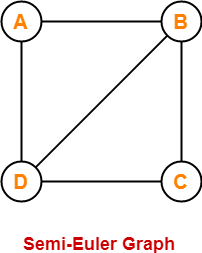Here,

• This graph contains an Euler trail BCDBAD.
• But it does not contain an Euler circuit.
• Therefore, it is a semi-Euler graph.

## Important Notes-

### Note-01:

To check whether any graph is an Euler graph or not, any one of the following two ways may be used-

• If the graph is connected and contains an Euler circuit, then it is an Euler graph.
• If all the vertices of the graph are of even degree, then it is an Euler graph.

### Note-02:

To check whether any graph contains an Euler circuit or not,

• Just make sure that all its vertices are of even degree.
• If all its vertices are of even degree, then graph contains an Euler circuit otherwise not.

### Note-03:

To check whether any graph is a semi-Euler graph or not,

• Just make sure that it is connected and contains an Euler trail.
• If the graph is connected and contains an Euler trail, then graph is a semi-Euler graph otherwise not.

### Note-04:

To check whether any graph contains an Euler trail or not,

• Just make sure that the number of vertices in the graph with odd degree are not more than 2.
• If the number of vertices with odd degree are at most 2, then graph contains an Euler trail otherwise not.

### Note-05:

• A graph will definitely contain an Euler trail if it contains an Euler circuit.
• A graph may or may not contain an Euler circuit if it contains an Euler trail.

### Note-06:

• An Euler graph is definitely be a semi-Euler graph.
• But a semi-Euler graph may or may not be an Euler graph.

## Problems-

Which of the following is / are Euler Graphs?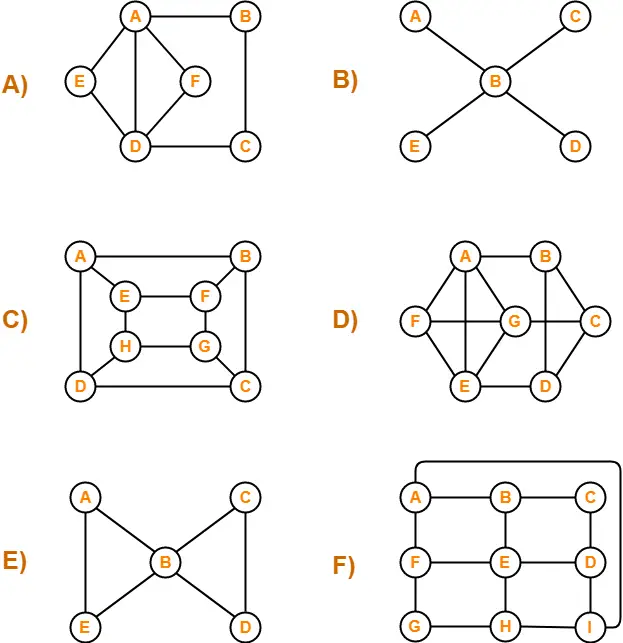## Solutions-

If all the vertices of a graph are of even degree, then graph is an Euler Graph otherwise not.

Using the above rule, we have-

A) It is an Euler graph.

B) It is not an Euler graph.

C) It is not an Euler graph.

D) It is not an Euler graph.

E) It is an Euler graph.

F) It is not an Euler graph.

To gain better understanding about Euler Graphs in Graph Theory,

Watch this Video Lecture

Next Article- Hamiltonian Graph

Get more notes and other study material of Graph Theory.

Watch video lectures by visiting our YouTube channel LearnVidFun.

## Chromatic Number-

Before you go through this article, make sure that you have gone through the previous article on Chromatic Number.

We gave discussed-

• Graph Coloring is a process of assigning colors to the vertices of a graph.
• It ensures that no two adjacent vertices of the graph are colored with the same color.
• Chromatic Number is the minimum number of colors required to properly color any graph.

In this article, we will discuss how to find Chromatic Number of any graph.

## Graph Coloring Algorithm-

• There exists no efficient algorithm for coloring a graph with minimum number of colors.
• Graph Coloring is a NP complete problem.

However, a following greedy algorithm is known for finding the chromatic number of any given graph.

## Greedy Algorithm-

### Step-01:

Color first vertex with the first color.

### Step-02:

Now, consider the remaining (V-1) vertices one by one and do the following-

• Color the currently picked vertex with the lowest numbered color if it has not been used to color any of its adjacent vertices.
• If it has been used, then choose the next least numbered color.
• If all the previously used colors have been used, then assign a new color to the currently picked vertex.

## Drawbacks of Greedy Algorithm-

There are following drawbacks of the above Greedy Algorithm-

• The above algorithm does not always use minimum number of colors.
• The number of colors used sometimes depend on the order in which the vertices are processed.

Also Read- Types of Graphs in Graph Theory

## Problem-01:

Find chromatic number of the following graph-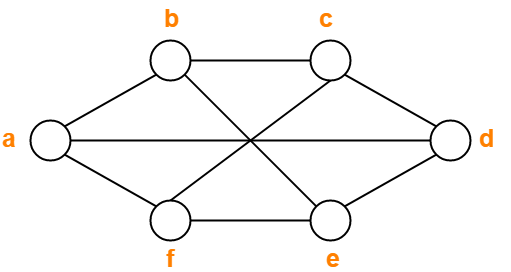## Solution-

Applying Greedy Algorithm, we have-

 Vertex a b c d e f Color C1 C2 C1 C2 C1 C2

From here,

• Minimum number of colors used to color the given graph are 2.
• Therefore, Chromatic Number of the given graph = 2.

The given graph may be properly colored using 2 colors as shown below-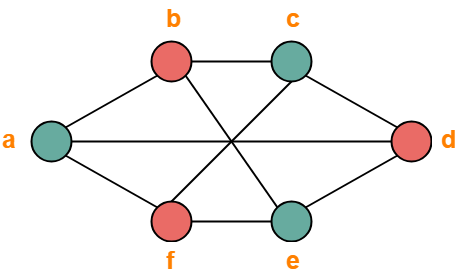## Problem-02:

Find chromatic number of the following graph-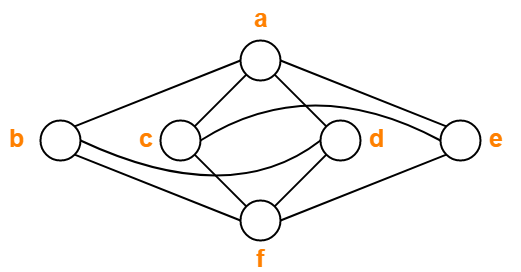## Solution-

Applying Greedy Algorithm, we have-

 Vertex a b c d e f Color C1 C2 C2 C3 C3 C1

From here,

• Minimum number of colors used to color the given graph are 3.
• Therefore, Chromatic Number of the given graph = 3.

The given graph may be properly colored using 3 colors as shown below-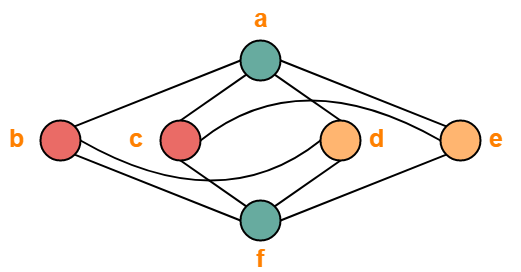## Problem-03:

Find chromatic number of the following graph-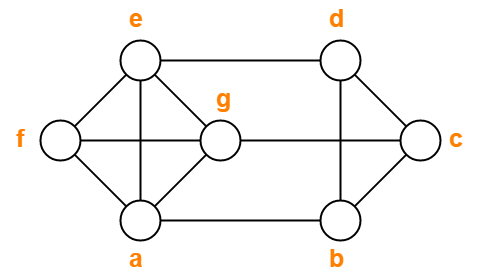## Solution-

Applying Greedy Algorithm, we have-

 Vertex a b c d e f g Color C1 C2 C1 C3 C2 C3 C4

From here,

• Minimum number of colors used to color the given graph are 4.
• Therefore, Chromatic Number of the given graph = 4.

The given graph may be properly colored using 4 colors as shown below-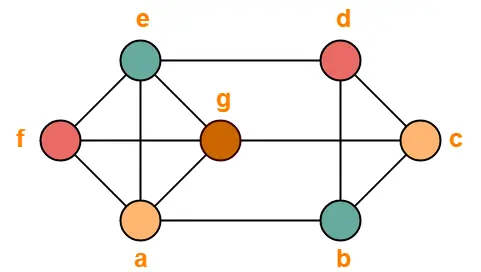## Problem-04:

Find chromatic number of the following graph-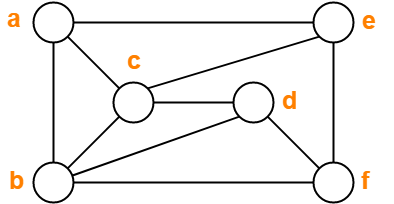## Solution-

Applying Greedy Algorithm, we have-

 Vertex a b c d e f Color C1 C2 C3 C1 C2 C3

From here,

• Minimum number of colors used to color the given graph are 3.
• Therefore, Chromatic Number of the given graph = 3.

The given graph may be properly colored using 3 colors as shown below-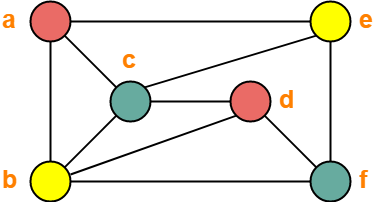## Problem-05:

Find chromatic number of the following graph-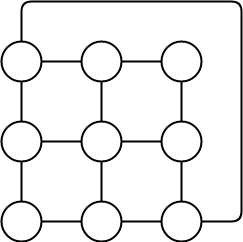## Solution-

Applying Greedy Algorithm,

• Minimum number of colors required to color the given graph are 3.
• Therefore, Chromatic Number of the given graph = 3.

The given graph may be properly colored using 3 colors as shown below-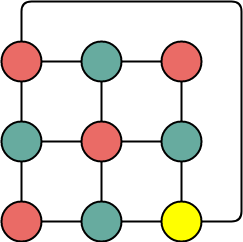To gain better understanding about How to Find Chromatic Number,

Watch this Video Lecture

Get more notes and other study material of Graph Theory.

Watch video lectures by visiting our YouTube channel LearnVidFun.

## Graph Coloring-

 Graph Coloring is a process of assigning colors to the vertices of a graphsuch that no two adjacent vertices of it are assigned the same color.

• Graph Coloring is also called as Vertex Coloring.
• It ensures that there exists no edge in the graph whose end vertices are colored with the same color.
• Such a graph is called as a Properly colored graph.

### Graph Coloring Example-

The following graph is an example of a properly colored graph-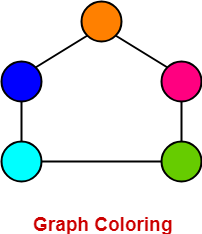In this graph,

• No two adjacent vertices are colored with the same color.
• Therefore, it is a properly colored graph.

### Graph Coloring Applications-

Some important applications of graph coloring are as follows-

• Map Coloring
• Preparing Time Table
• Assignment
• Conflict Resolution
• Sudoku

## Chromatic Number-

 Chromatic Number is the minimum number of colors required to properly color any graph.ORChromatic Number is the minimum number of colors required to color any graphsuch that no two adjacent vertices of it are assigned the same color.

### Chromatic Number Example-

Consider the following graph-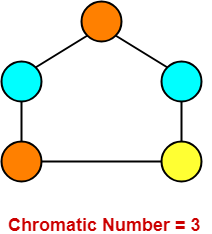In this graph,

• No two adjacent vertices are colored with the same color.
• Minimum number of colors required to properly color the vertices = 3.
• Therefore, Chromatic number of this graph = 3.
• We can not properly color this graph with less than 3 colors.

Also Read- Types of Graphs in Graph Theory

## Chromatic Number Of Graphs-

Chromatic Number of some common types of graphs are as follows-

## 1. Cycle Graph-

• A simple graph of ‘n’ vertices (n>=3) and ‘n’ edges forming a cycle of length ‘n’ is called as a cycle graph.
• In a cycle graph, all the vertices are of degree 2.

### Chromatic Number

• If number of vertices in cycle graph is even, then its chromatic number = 2.
• If number of vertices in cycle graph is odd, then its chromatic number = 3.

### Examples-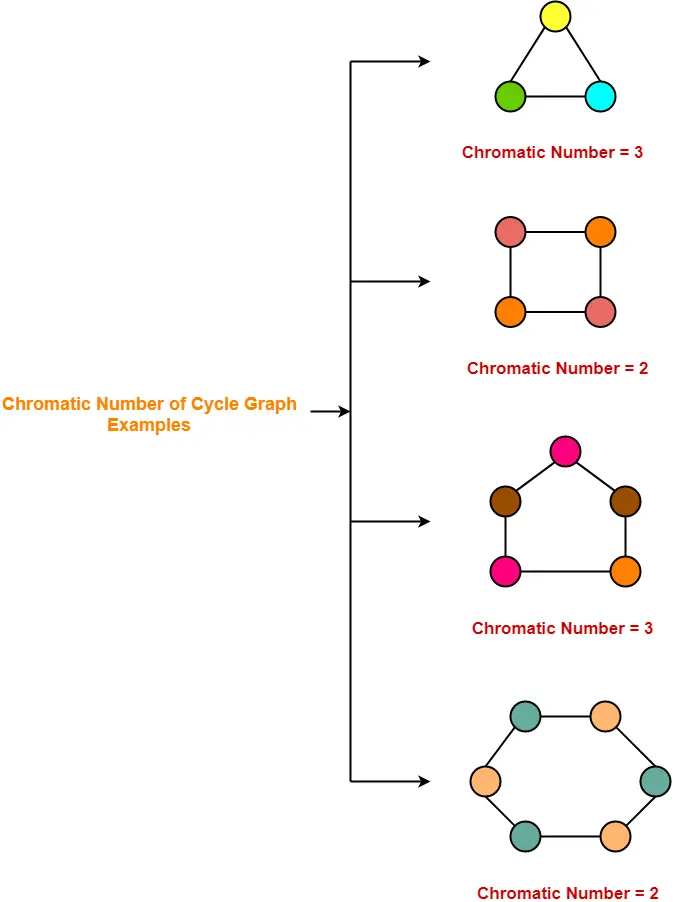## 2. Planar Graphs-

A Planar Graph is a graph that can be drawn in a plane such that none of its edges cross each other.

### Chromatic Number

Chromatic Number of any Planar Graph

= Less than or equal to 4

### Examples-

• All the above cycle graphs are also planar graphs.
• Chromatic number of each graph is less than or equal to 4.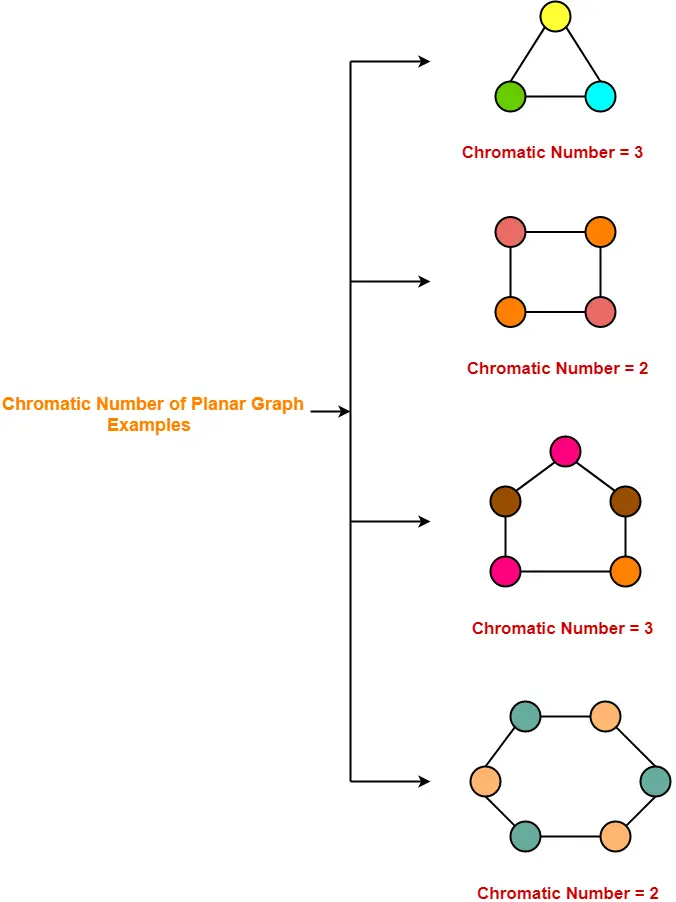## 3. Complete Graphs-

• A complete graph is a graph in which every two distinct vertices are joined by exactly one edge.
• In a complete graph, each vertex is connected with every other vertex.
• So to properly it, as many different colors are needed as there are number of vertices in the given graph.

### Chromatic Number

Chromatic Number of any Complete Graph

= Number of vertices in that Complete Graph

### Examples-## 4. Bipartite Graphs-

• A Bipartite Graph consists of two sets of vertices X and Y.
• The edges only join vertices in X to vertices in Y, not vertices within a set.

### Chromatic Number

Chromatic Number of any Bipartite Graph

= 2

### Example-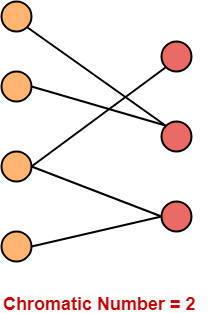## 5. Trees-

• A Tree is a special type of connected graph in which there are no circuits.
• Every tree is a bipartite graph.
• So, chromatic number of a tree with any number of vertices = 2.

### Chromatic Number

Chromatic Number of any tree

= 2

### Examples-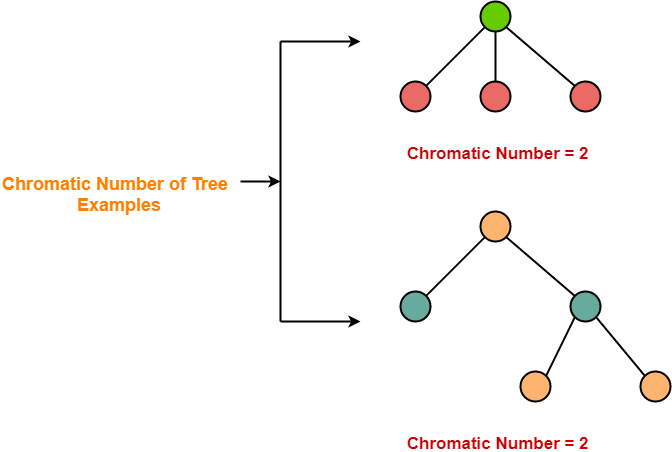To gain better understanding about Graph Coloring & Chromatic Number,

Watch this Video Lecture

Next Article- Graph Coloring Algorithm

Get more notes and other study material of Graph Theory.

Watch video lectures by visiting our YouTube channel LearnVidFun.

## Types of Graphs-

Before you go through this article, make sure that you have gone through the previous article on various Types of Graphs in Graph Theory.

We have discussed-

• A graph is a collection of vertices connected to each other through a set of edges.
• The study of graphs is known as Graph Theory.## Planar Graph-

A planar graph may be defined as-

 In graph theory,Planar graph is a graph that can be drawn in a plane such that none of its edges cross each other.

### Planar Graph Example-

The following graph is an example of a planar graph-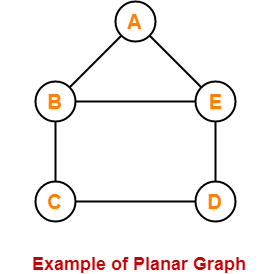Here,

• In this graph, no two edges cross each other.
• Therefore, it is a planar graph.

## Regions of Plane-

The planar representation of the graph splits the plane into connected areas called as Regions of the plane.

Each region has some degree associated with it given as-

• Degree of Interior region = Number of edges enclosing that region
• Degree of Exterior region = Number of edges exposed to that region

### Example-

Consider the following planar graph-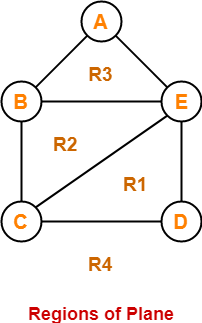Here, this planar graph splits the plane into 4 regions- R1, R2, R3 and R4 where-

• Degree (R1) = 3
• Degree (R2) = 3
• Degree (R3) = 3
• Degree (R4) = 5

## Planar Graph Chromatic Number-

• Chromatic Number of any planar graph is always less than or equal to 4.
• Thus, any planar graph always requires maximum 4 colors for coloring its vertices.

## Planar Graph Properties-

### Property-01:

In any planar graph, Sum of degrees of all the vertices = 2 x Total number of edges in the graph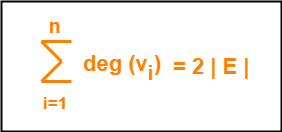### Property-02:

In any planar graph, Sum of degrees of all the regions = 2 x Total number of edges in the graph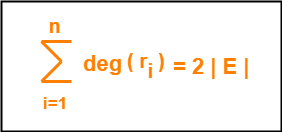### Case-01:

In any planar graph, if degree of each region is K, then-

 K x |R| = 2 x |E|

### Case-02:

In any planar graph, if degree of each region is at least K (>=K), then-

 K x |R| <= 2 x |E|

### Case-03:

In any planar graph, if degree of each region is at most K (<=K), then-

 K x |R| >= 2 x |E|

### Property-03:

If G is a connected planar simple graph with ‘e’ edges, ‘v’ vertices and ‘r’ number of regions in the planar representation of G, then-

 r = e – v + 2

This is known as Euler’s Formula.

It remains same in all the planar representations of the graph.

### Property-04:

If G is a planar graph with k components, then-

 r = e – v + (k + 1)

## Problem-01:

Let G be a connected planar simple graph with 25 vertices and 60 edges. Find the number of regions in G.

## Solution-

Given-

• Number of vertices (v) = 25
• Number of edges (e) = 60

By Euler’s formula, we know r = e – v + 2.

Substituting the values, we get-

Number of regions (r)

= 60 – 25 + 2

= 37

Thus, Total number of regions in G = 37.

## Problem-02:

Let G be a planar graph with 10 vertices, 3 components and 9 edges. Find the number of regions in G.

## Solution-

Given-

• Number of vertices (v) = 10
• Number of edges (e) = 9
• Number of components (k) = 3

By Euler’s formula, we know r = e – v + (k+1).

Substituting the values, we get-

Number of regions (r)

= 9 – 10 + (3+1)

= -1 + 4

= 3

Thus, Total number of regions in G = 3.

## Problem-03:

Let G be a connected planar simple graph with 20 vertices and degree of each vertex is 3. Find the number of regions in G.

## Solution-

Given-

• Number of vertices (v) = 20
• Degree of each vertex (d) = 3

### Calculating Total Number Of Edges (e)-

By sum of degrees of vertices theorem, we have-

Sum of degrees of all the vertices = 2 x Total number of edges

Number of vertices x Degree of each vertex = 2 x Total number of edges

20 x 3 = 2 x e

∴ e = 30

Thus, Total number of edges in G = 30.

### Calculating Total Number Of Regions (r)-

By Euler’s formula, we know r = e – v + 2.

Substituting the values, we get-

Number of regions (r)

= 30 – 20 + 2

= 12

Thus, Total number of regions in G = 12.

## Problem-04:

Let G be a connected planar simple graph with 35 regions, degree of each region is 6. Find the number of vertices in G.

## Solution-

Given-

• Number of regions (n) = 35
• Degree of each region (d) = 6

### Calculating Total Number Of Edges (e)-

By sum of degrees of regions theorem, we have-

Sum of degrees of all the regions = 2 x Total number of edges

Number of regions x Degree of each region = 2 x Total number of edges

35 x 6 = 2 x e

∴ e = 105

Thus, Total number of edges in G = 105.

### Calculating Total Number Of Vertices (v)-

By Euler’s formula, we know r = e – v + 2.

Substituting the values, we get-

35 = 105 – v + 2

∴ v = 72

Thus, Total number of vertices in G = 72.

## Problem-05:

Let G be a connected planar graph with 12 vertices, 30 edges and degree of each region is k. Find the value of k.

## Solution-

Given-

• Number of vertices (v) = 12
• Number of edges (e) = 30
• Degree of each region (d) = k

### Calculating Total Number Of Regions (r)-

By Euler’s formula, we know r = e – v + 2.

Substituting the values, we get-

Number of regions (r)

= 30 – 12 + 2

= 20

Thus, Total number of regions in G = 20.

### Calculating Value Of k-

By sum of degrees of regions theorem, we have-

Sum of degrees of all the regions = 2 x Total number of edges

Number of regions x Degree of each region = 2 x Total number of edges

20 x k = 2 x 30

∴ k = 3

Thus, Degree of each region in G = 3.

## Problem-06:

What is the maximum number of regions possible in a simple planar graph with 10 edges?

## Solution-

In a simple planar graph, degree of each region is >= 3.

So, we have 3 x |R| <= 2 x |E|.

Substituting the value |E| = 10, we get-

3 x |R| <= 2 x 10

|R| <= 6.67

|R| <= 6

Thus, Maximum number of regions in G = 6.

## Problem-07:

What is the minimum number of edges necessary in a simple planar graph with 15 regions?

## Solution-

In a simple planar graph, degree of each region is >= 3.

So, we have 3 x |R| <= 2 x |E|.

Substituting the value |R| = 15, we get-

3 x 15 <= 2 x |E|

|E| >= 22.5

|E| >= 23

Thus, Minimum number of edges required in G = 23.

To gain better understanding about Planar Graphs in Graph Theory,

Watch this Video Lecture

Next Article- Euler Graph

Get more notes and other study material of Graph Theory.

Watch video lectures by visiting our YouTube channel LearnVidFun.

## Walk in Graph Theory-

In graph theory,

• A walk is defined as a finite length alternating sequence of vertices and edges.
• The total number of edges covered in a walk is called as Length of the Walk.

### Walk in Graph Theory Example-

Consider the following graph-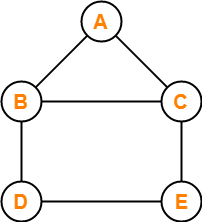In this graph, few examples of walk are-

• a , b , c , e , d                    (Length = 4)
• d , b , a , c , e , d , e , c     (Length = 7)
• e , c , b , a , c , e , d          (Length = 6)

## Open Walk in Graph Theory-

In graph theory, a walk is called as an Open walk if-

• Length of the walk is greater than zero
• And the vertices at which the walk starts and ends are different.

## Closed Walk in Graph Theory-

In graph theory, a walk is called as a Closed walk if-

• Length of the walk is greater than zero
• And the vertices at which the walk starts and ends are same.

### NOTE

It is important to note the following points-

• If length of the walk = 0, then it is called as a Trivial Walk.
• Both vertices and edges can repeat in a walk whether it is an open walk or a closed walk.

## Path in Graph Theory-

In graph theory, a path is defined as an open walk in which-

• Neither vertices (except possibly the starting and ending vertices) are allowed to repeat.
• Nor edges are allowed to repeat.

## Cycle in Graph Theory-

In graph theory, a cycle is defined as a closed walk in which-

• Neither vertices (except possibly the starting and ending vertices) are allowed to repeat.
• Nor edges are allowed to repeat.

OR

In graph theory, a closed path is called as a cycle.

## Trail in Graph Theory-

In graph theory, a trail is defined as an open walk in which-

• Vertices may repeat.
• But edges are not allowed to repeat.

## Circuit in Graph Theory-

In graph theory, a circuit is defined as a closed walk in which-

• Vertices may repeat.
• But edges are not allowed to repeat.

OR

In graph theory, a closed trail is called as a circuit.

### NOTE

It is important to note the following points-

• Every path is a trail but every trail need not be a path.
• Every cycle is a circuit but every circuit need not be a cycle.
• For directed graphs, we put term “directed” in front of all the terms defined above.

## Important Chart-

The following chart summarizes the above definitions and is helpful in remembering them-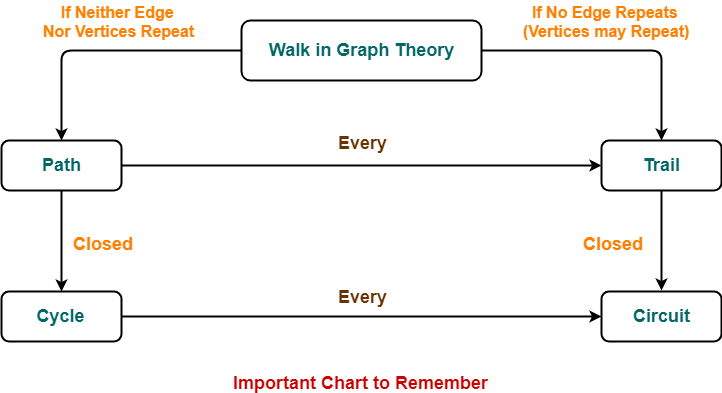Also Read- Types of Graphs in Graph Theory

## Problem-01:

Consider the following graph-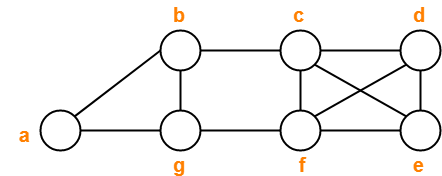Decide which of the following sequences of vertices determine walks.

For those that are walks, decide whether it is a circuit, a path, a cycle or a trail.

1. a , b , g , f , c , b
2. b , g , f , c , b , g , a
3. c , e , f , c
4. c , e , f , c , e
5. a , b , f , a
6. f , d , e , c , b

1. Trail
2. Walk
3. Cycle
4. Walk
5. Not a walk
6. Path

## Problem-02:

Consider the following graph-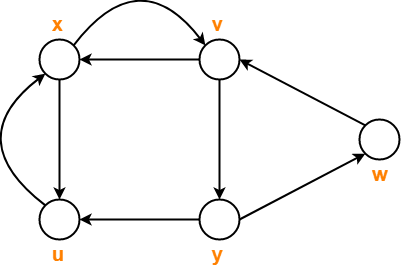Consider the following sequences of vertices and answer the questions that follow-

1. x , v , y , w , v
2. x , u , x , u , x
3. x , u , v , y , x
4. x , v , y , w , v , u , x

1. Which of the above given sequences are directed walks?
2. What are the lengths of directed walks?
3. Which directed walks are also directed paths?
4. Which directed walks are also directed cycles?

## Solution-

### Part-01:

• Only (A) and (B) are the directed walks.
• (C) is not a directed walk since there exists no arc from vertex u to vertex v.
• (D) is not a directed walk since there exists no arc from vertex v to vertex u.

### Part-02:

Both the directed walks (A) and (B) have length = 4.

### Part-03:

• Neither (A) nor (B) are directed paths.
• This is because vertices repeat in both of them.
• Vertex v repeats in Walk (A) and vertex u repeats in walk (B).

### Part-04:

• Neither of them are directed cycles.
• Walk (A) does not represent a directed cycle because its starting and ending vertices are not same.
• Walk (B) does not represent a directed cycle because it repeats vertices/edges.

## Problem-03:

Consider the following graph-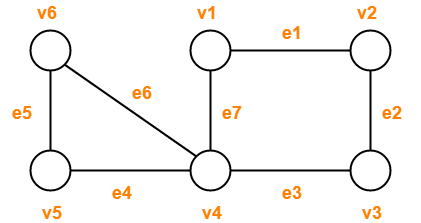Observe the given sequences and predict the nature of walk in each case-

1. v1e1v2e2v3e2v2
2. v4e7v1e1v2e2v3e3v4e4v5
3. v1e1v2e2v3e3v4e4v5
4. v1e1v2e2v3e3v4e7v1
5. v6e5v5e4v4e3v3e2v2e1v1e7v4e6v6

## Solution-

1. Open walk
2. Trail (Not a path because vertex v4 is repeated)
3. Path
4. Cycle
5. Circuit (Not a cycle because vertex v4 is repeated)

To gain better understanding about Walk in Graph Theory,

Watch this Video Lecture

Next Article- Graph Coloring

Get more notes and other study material of Graph Theory.

Watch video lectures by visiting our YouTube channel LearnVidFun.